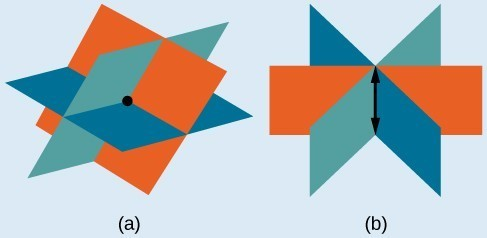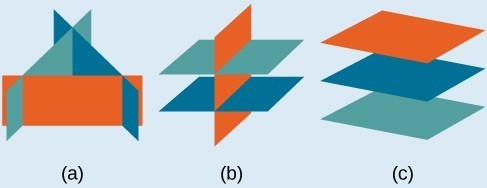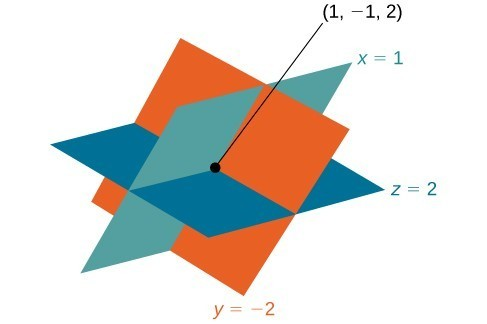## Solving Systems of Three Equations in Three Variables

In order to solve systems of equations in three variables, known as three-by-three systems, the primary tool we will be using is called Gaussian elimination, named after the prolific German mathematician Karl Friedrich Gauss. While there is no definitive order in which operations are to be performed, there are specific guidelines as to what type of moves can be made. We may number the equations to keep track of the steps we apply. The goal is to eliminate one variable at a time to achieve upper triangular form, the ideal form for a three-by-three system because it allows for straightforward back-substitution to find a solution $\left(x,y,z\right),\text{}$ which we call an ordered triple. A system in upper triangular form looks like the following:

$\begin{array}{l}Ax+By+Cz=D\hfill \\ \text{ }Ey+Fz=G\hfill \\ \text{ }Hz=K\hfill \end{array}$

The third equation can be solved for $z,\text{}$ and then we back-substitute to find $y$ and $x$. To write the system in upper triangular form, we can perform the following operations:

1. Interchange the order of any two equations.
2. Multiply both sides of an equation by a nonzero constant.
3. Add a nonzero multiple of one equation to another equation.

The solution set to a three-by-three system is an ordered triple $\left\{\left(x,y,z\right)\right\}$. Graphically, the ordered triple defines the point that is the intersection of three planes in space. You can visualize such an intersection by imagining any corner in a rectangular room. A corner is defined by three planes: two adjoining walls and the floor (or ceiling). Any point where two walls and the floor meet represents the intersection of three planes.

### A General Note: Number of Possible Solutions

Figure 2 and Figure 3 illustrate possible solution scenarios for three-by-three systems.

• Systems that have a single solution are those which, after elimination, result in a solution set consisting of an ordered triple $\left\{\left(x,y,z\right)\right\}$. Graphically, the ordered triple defines a point that is the intersection of three planes in space.
• Systems that have an infinite number of solutions are those which, after elimination, result in an expression that is always true, such as $0=0$. Graphically, an infinite number of solutions represents a line or coincident plane that serves as the intersection of three planes in space.
• Systems that have no solution are those that, after elimination, result in a statement that is a contradiction, such as $3=0$. Graphically, a system with no solution is represented by three planes with no point in common.Figure 2. (a)Three planes intersect at a single point, representing a three-by-three system with a single solution. (b) Three planes intersect in a line, representing a three-by-three system with infinite solutions.Figure 3

### Example 1: Determining Whether an Ordered Triple Is a Solution to a System

Determine whether the ordered triple $\left(3,-2,1\right)$ is a solution to the system.

$\begin{array}{l}\text{ }x+y+z=2\hfill \\ 6x - 4y+5z=31\hfill \\ 5x+2y+2z=13\hfill \end{array}$

### Solution

We will check each equation by substituting in the values of the ordered triple for $x,y$, and $z$.

$\begin{array}{ccccc}\begin{array}{r}\hfill x+y+z=2\\ \hfill \left(3\right)+\left(-2\right)+\left(1\right)=2\\ \hfill \text{True}\end{array}& & \begin{array}{r}\hfill \text{}6x - 4y+5z=31\\ \hfill 6\left(3\right)-4\left(-2\right)+5\left(1\right)=31\\ \hfill 18+8+5=31\\ \hfill \text{True}\end{array}& & \begin{array}{r}\hfill \text{}5x+2y+2z=13\\ \hfill 5\left(3\right)+2\left(-2\right)+2\left(1\right)=13\\ \hfill \text{}15 - 4+2=13\\ \hfill \text{True}\end{array}\end{array}$

The ordered triple $\left(3,-2,1\right)$ is indeed a solution to the system.

### How To: Given a linear system of three equations, solve for three unknowns.

1. Pick any pair of equations and solve for one variable.
2. Pick another pair of equations and solve for the same variable.
3. You have created a system of two equations in two unknowns. Solve the resulting two-by-two system.
4. Back-substitute known variables into any one of the original equations and solve for the missing variable.

### Example 2: Solving a System of Three Equations in Three Variables by Elimination

Find a solution to the following system:

$\begin{array}{ll}\text{ }x - 2y+3z=9\hfill & \text{(1)}\hfill \\ \text{ }-x+3y-z=-6\hfill & \text{(2)}\hfill \\ 2x - 5y+5z=17\hfill & \text{(3)}\hfill \end{array}$

### Solution

There will always be several choices as to where to begin, but the most obvious first step here is to eliminate $x$ by adding equations (1) and (2).

$\frac{\begin{array}{ll}\text{ }\text{}x - 2y+3z=9\hfill & \text{(1)}\hfill \\ \text{ }-x+3y-z=-6\hfill & \text{ (2)}\hfill \end{array}}{\begin{array}{ll}\text{ }\text{}\text{}y+2z=3\hfill & \text{ (3)}\hfill \end{array}}$

The second step is multiplying equation (1) by $-2$ and adding the result to equation (3). These two steps will eliminate the variable $x$.

$\begin{array} \hfill−2x+4y−6z=−18 \hfill& \left(1\right)\text{ multiplied by }−2 \\ 2x−5y+5z=17 \hfill& \left(3\right) \\ \text{_____________________________} \\ \hfill−y−z=−1\left(5\right)\hfill& \end{array}$

In equations (4) and (5), we have created a new two-by-two system. We can solve for $z$ by adding the two equations.

$\frac{\begin{array}{l}\begin{array}{l}\hfill \\ \text{}y+2z=3\text{ }\left(4\right)\hfill \end{array}\hfill \\ -y-z=-1\text{ }\left(5\right)\hfill \end{array}}{z=2\text{ }\left(6\right)}$

Choosing one equation from each new system, we obtain the upper triangular form:

$\begin{array}{ll}\text{}\text{}x - 2y+3z=9\text{ }\hfill & \left(1\right)\hfill \\ \text{ }y+2z=3\hfill & \left(4\right)\hfill \\ \text{ }z=2\hfill & \left(6\right)\hfill \end{array}$

Next, we back-substitute $z=2$ into equation (4) and solve for $y$.

$\begin{array}{l}y+2\left(2\right)=3\hfill \\ \text{ }y+4=3\hfill \\ \text{ }y=-1\hfill \end{array}$

Finally, we can back-substitute $z=2$ and $y=-1$ into equation (1). This will yield the solution for $x$.

$\begin{array}{r}\hfill x - 2\left(-1\right)+3\left(2\right)=9\\ \hfill \text{ }x+2+6=9\\ \hfill \text{ }x=1\end{array}$

The solution is the ordered triple $\left(1,-1,2\right)$.Figure 4

In the problem posed at the beginning of the section, John invested his inheritance of $12,000 in three different funds: part in a money-market fund paying 3% interest annually; part in municipal bonds paying 4% annually; and the rest in mutual funds paying 7% annually. John invested$4,000 more in mutual funds than he invested in municipal bonds. The total interest earned in one year was $670. How much did he invest in each type of fund? ### Solution To solve this problem, we use all of the information given and set up three equations. First, we assign a variable to each of the three investment amounts: $\begin{array}{l}x=\text{amount invested in money-market fund}\hfill \\ y=\text{amount invested in municipal bonds}\hfill \\ z=\text{amount invested in mutual funds}\hfill \end{array}$ The first equation indicates that the sum of the three principal amounts is$12,000.

$x+y+z=12,000$

We form the second equation according to the information that John invested $4,000 more in mutual funds than he invested in municipal bonds. $z=y+4,000$ The third equation shows that the total amount of interest earned from each fund equals$670.

$0.03x+0.04y+0.07z=670$

Then, we write the three equations as a system.

$\begin{array}{l}\text{ }x+y+z=12,000\hfill \\ \text{ }-y+z=4,000\hfill \\ 0.03x+0.04y+0.07z=670\hfill \end{array}$

To make the calculations simpler, we can multiply the third equation by 100. Thus,

$\begin{array}{ll}\text{ }x+\text{ }y+z\text{ }=12,000\hfill & \left(1\right)\hfill \\ \text{ }-y+z\text{ }=4,000\hfill & \left(2\right)\hfill \\ 3x+4y+7z=67,000\hfill & \left(3\right)\hfill \end{array}$

Step 1. Interchange equation (2) and equation (3) so that the two equations with three variables will line up.

$\begin{array}{l}\text{ }x+\text{ }y +\text{ }z=12,000\hfill \\ 3x+4y +7z=67,000\hfill \\ \text{ }-y\text{ }+\text{ }z=4,000\hfill \end{array}$

Step 2. Multiply equation (1) by $-3$ and add to equation (2). Write the result as row 2.

$\begin{array}{l}x+y+z\text{ }=12,000\hfill \\ \text{ }y+4z=31,000\hfill \\ \text{ }-y+z\text{ }=4,000\hfill \end{array}$

Step 3. Add equation (2) to equation (3) and write the result as equation (3).

$\begin{array}{l}x+y+\text{ }z=12,000\hfill \\ \text{ }y+4z=31,000\hfill \\ \text{ }5z\text{ }=35,000\hfill \end{array}$

Step 4. Solve for $z$ in equation (3). Back-substitute that value in equation (2) and solve for $y$. Then, back-substitute the values for $z$ and $y$ into equation (1) and solve for $x$.

$\begin{array}{l}\text{ }5z=35,000\hfill \\ \text{ }z=7,000\hfill \\ \hfill \\ \hfill \\ \text{ }y+4\left(7,000\right)=31,000\hfill \\ \text{ }y=3,000\hfill \\ \hfill \\ \hfill \\ x+3,000+7,000=12,000\hfill \\ \text{ }x=2,000\hfill \end{array}$

John invested $2,000 in a money-market fund,$3,000 in municipal bonds, and \$7,000 in mutual funds.

### Try It 1

Solve the system of equations in three variables.

$\begin{array}{l}2x+y - 2z=-1\hfill \\ 3x - 3y-z=5\hfill \\ x - 2y+3z=6\hfill \end{array}$

Solution